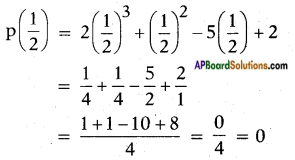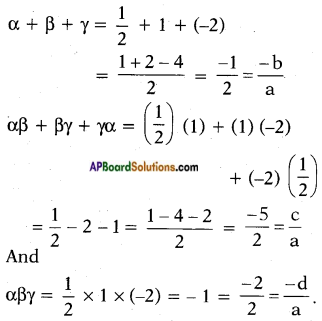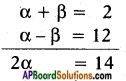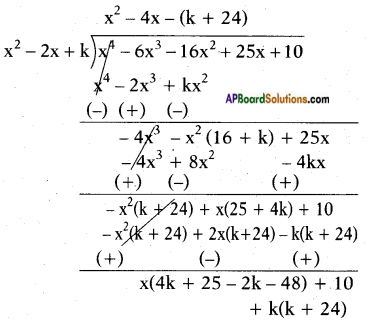AP State Board Syllabus AP SSC 10th Class Maths Textbook Solutions Chapter 3 Polynomials Optional Exercise Textbook Questions and Answers.

## AP State Syllabus SSC 10th Class Maths Solutions 3rd Lesson Polynomials Optional Exercise

### 10th Class Maths 3rd Lesson Polynomials Optional Exercise Textbook Questions and Answers

Question 1.
Verify that the numbers given along-side the cubic polynomials below are their zeroes. Also verify the relation-ship between the zeroes and the coefficients in each case:
i) 2x3 + x2 – 5x + 2; ($$\frac{1}{2}$$, 1,-2)
ii) x3 + 4x2 + 5x – 2 ; (1, 1, 1)
i) Given polynomial 2x3 + x2 – 5x + 2
Comparing the given polynomial with ax3 + bx2 + cx + d,
we get a = 2, b = 1, c = – 5 and d = 2P(1) = 2(1)3 + (1)2 – 5(1) – 2
= 2 + 1 – 5 + 2 = 0
p(-2) = 2(-2)3 + (-2)2 – 5(-2) + 2
= 2(-8) + 4 + 10 + 2
= – 16 + 16 = 0
∴ $$\frac{1}{2}$$, 1 and – 2 are the zeroes of 2x3 + x2 – 5x + 2
So, α = $$\frac{1}{2}$$, β = 1 and γ = – 2 Therefore,ii) Given polynomial x3 + 4x2 + 5x – 2
Comparing the given polynomial with ax3 + bx2 + cx + d,
we get a = 1, b = 4, c = 5 and d = – 2.
Given zeroes are (1, 1, 1)
p(1) = (1)3 + 4(1)2 + 5(1) – 2
= 1 + 4 + 5 – 2
= 10 – 2 = 8
∴ (1, 1, 1) are not zeroes of the given polynomial p(x).

Question 2.
Find a cubic polynomial with the sum, sum of the product of its zeroes taken two at a time, and the product of its zeroes as 2, -7, -14 respectively.
Let the cubic polynomial be
ax3 + bx2 + cx + d, and its zeroes be α, β and γ.
Then,
α + β + γ = 2 = $$\frac{-(-2)}{1}$$ = $$\frac{-b}{a}$$
αβ + βγ + γα = -7 = $$\frac{-7}{1}$$ = $$\frac{c}{a}$$
αβγ = – 14 = $$\frac{-14}{1}$$ = $$\frac{-d}{a}$$
a = 1, then b = -2, c = -7 and d = 14.
So, one cubic polynomial which satisfies the given conditions will be x3 – 2x2 – 7x + 14.

Question 3.
If the zeroes of the polynomial x3 – 3x2 + x + 1 are a – b, a, a + b, find a and b.
Given polynomial x3 – 3x2 + x + 1
Since, (a – b), a, (a + b) are the zeroes of the polynomial x3 – 3x2 + x + 1.
Therefore, sum of the zeroes
= (a – b) + a + (a + b) = $$\frac{-(-3)}{1}$$ = 3
So, 3a = 3 ⇒ a = 1
∴ Sum of the products of its zeroes taken two at a time.
= a(a – b) + a(a + b) + (a + b) (a – b) = $$\frac{1}{1}$$ = 1
⇒ a2 – ab + a2 + ab + a2 – b2 = 1
⇒ 3a2 – b2 = 1
So, 3(1)2 – b2 = 1 ⇒ 3 – b2 = 1
⇒ b2 = 2
⇒ b = √2 = ± √2
Here, a = 1 and b = ± √2Question 4.
If two zeroes of the polynomial x4 – 6x3 – 26x2 + 138x – 35 are 2 ± √3 , find other zeroes.
Let the other, two zeroes are α, β.
Then the sum of zeroes of given polynomial = 2 + √3 + 2 – √3 + α + β
= $$\frac{-b}{a}$$ = $$\frac{-(-6)}{1}$$ = 6
4 + α + β = 6
⇒ α + β = 2 ….. (1)
Now product of zeroes is
(2 + √3 ) (2 – √3) (α) (β)= $$\frac{e}{a}$$ = $$\frac{-35}{1}$$
(4 – 3) (αβ) = – 35
⇒ αβ = – 35 …… (2)
Now (α – β)2 = (α + β)2 – 4αβ
= (2)2 – 4(-35) = 4 + 140 = 144
⇒ (α – β) = ± 12 ….. (3)
Now solving (1) & (3) we get⇒ α = 7; then α + β = 7 + β = 2
⇒ β = -5
The remaining zeroes are α, β
= 7, -5
So total zeroes of given polynomial are 2 + √3, 2 – √3, 7, – 5.Question 5.
If the polynomial x4 – 6x3 – 16x2 + 25x + 10 is divided by another polynomial x2 – 2x + k, the remainder comes out to be x + a, find k and a.
Given polynomial x4 – 6x3 – 16x2 + 25x + 10 and another polynomial is x2 – 2x + k.
Remainder is x + a
Let us divide
x4 – 6x3 – 16x2 + 25x + 10 by x2 – 2x + k∴ Remainder
= x(4k + 25 – 2k – 48) + 10 + k(k + 24)
= x(2k – 23) + (k2 + 24k + 10)
Given remainder is x + a
on comparing the coefficients of x and constant terms on both sides
2k – 23 = 1 ……. (1)
2k = 1 + 23 = 24
⇒ k = $$\frac{24}{2}$$ = 12
k2 + 24k + 10 = a …….. (2)
Substitute ‘k’ value in equation (2)
(12)2 + 24(12) + 10 = a
144 + 288 + 10 = a
⇒ a = 442
∴ Required k = 12 and ‘a’ = 442